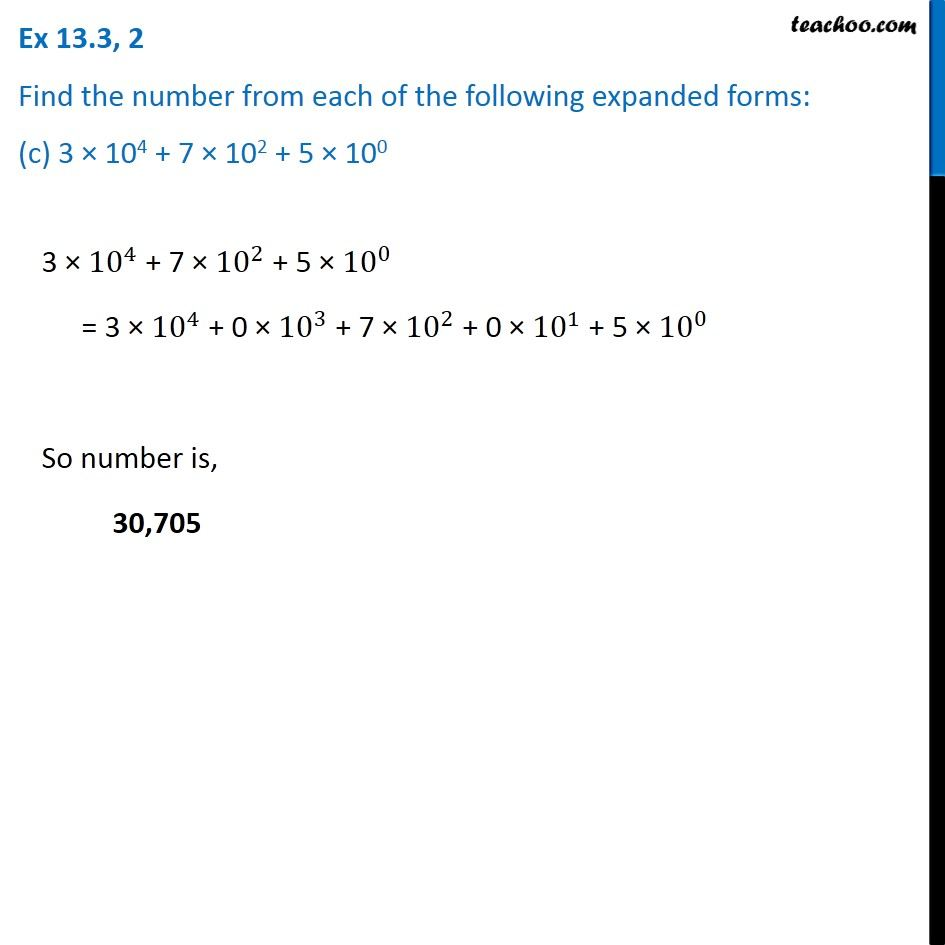Ex 13.3

Chapter 13 Class 7 Exponents and Powers
Serial order wiseIntroducing your new favourite teacher - Teachoo Black, at only ₹83 per month

### Transcript

Ex 13.3, 2 Find the number from each of the following expanded forms: (c) 3 × 104 + 7 × 102 + 5 × 100 3 × 〖10〗^4 + 7 × 〖10〗^2 + 5 × 〖10〗^0 = 3 × 〖10〗^4 + 0 × 〖10〗^3 + 7 × 〖10〗^2 + 0 × 〖10〗^1 + 5 × 〖10〗^0 So number is, 30,705